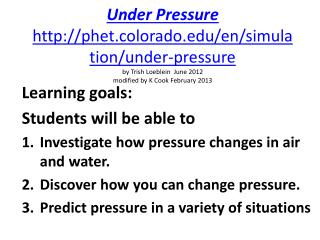DownloadDownload PresentationLearning goals: Students will be able to Investigate how pressure changes in air and water.

# Learning goals: Students will be able to Investigate how pressure changes in air and water.

Télécharger la présentation## Learning goals: Students will be able to Investigate how pressure changes in air and water.

- - - - - - - - - - - - - - - - - - - - - - - - - - - E N D - - - - - - - - - - - - - - - - - - - - - - - - - - -
##### Presentation Transcript

1. Under Pressurehttp://phet.colorado.edu/en/simulation/under-pressureby Trish Loeblein June 2012modified by K Cook February 2013 Learning goals: Students will be able to Investigate how pressure changes in air and water. Discover how you can change pressure. Predict pressure in a variety of situations

2. Directions: • Explore the simulation to find out how pressure changes in air and water. • Discover how you can change pressure in the simulation. • Go through the following slides as a guide and answer the questions. • Make your guess • Use the sim to check your guess • Go to the next slide to see if you got it right

3. 1. Order from lowest to highest pressure. • A<B<C • C<B<A • all are equal

5. 2. Look at the markers. A.Order from lowest to highest pressure. B. Why (think about F &SA)? X A. Y<Z<X B. Y<X<Z C. Z<X<Y D. X<Z<Y E. two are equal Y Z

6. answer B. Y<X<Z Y is less force because only air above (less F  less P) Z is most because even though same depth as X, it has less surface area (less SA  more P)

7. 3. What will happen to the pressure if more water is added?Is this related to force or surface area? • increase • decrease • stay the same

8. answer • increase because there is more force

9. 4. What will happen to the pressure if more water is added while the same amount is removed? Why? • increase • decrease • stay the same

10. answer C. stay the same because force is the same

11. 5. What will happen to the pressure if the fluid were changed to honey? Why? • increase • decrease • stay the same

12. answer • increase because more force

13. 6. If a 250 kg mass was put on the water column, what will happen to the pressure? Why? • increase • decrease • stay the same

14. answer • increase because more mass leads to more force (there is more weight)

15. 7. If the only change was to remove the air pressure , what will happen to the pressure? Why? • increase by 101.3 kPa • decrease by 101.3 kPa • stay the same • Something else

16. answer B. decrease by 101.3 kPa (If no air, then less force)

17. 8. If the only change was to go to a place where the gravity was doubled, what will happen to the pressure? Why? • Both pressures would double • Only the air pressure would double • The air pressure would double, and the water pressure would increase some • Something else

18. answer • Both pressures would double because increased gravity acting on the mass of air AND water is increasing the weight (force) of both (more gravity  more force  more pressure

19. 9. How do the pressures at the two locations compare? Why? • X>Y • Y>X • They are the same X Y

20. answer C. They are the same because it’s the surface area of the entire body of water and they are part of the same body of water